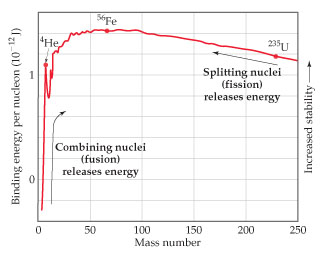# Problem: The atomic masses of hydrogen-2 (deuterium), helium-4, and lithium-6 are 2.014102 amu, 4.002602 amu, and 6.0151228 amu, respectively. For 4He, calculate the nuclear binding energy.

###### FREE Expert Solution

We are asked to calculate the nuclear binding energy for 4He.

To calculate for the nuclear binding energy:

Step 1: Determine the number of protons and neutrons

Step 2: Determine the total mass of protons and neutrons

Step 3: Calculate the mass defect (∆m)

Step 4: Determine nuclear binding energy per nucleon

80% (409 ratings)###### Problem Details

The atomic masses of hydrogen-2 (deuterium), helium-4, and lithium-6 are 2.014102 amu, 4.002602 amu, and 6.0151228 amu, respectively.For 4He, calculate the nuclear binding energy.

Frequently Asked Questions

What scientific concept do you need to know in order to solve this problem?

Our tutors have indicated that to solve this problem you will need to apply the Mass Defect concept. If you need more Mass Defect practice, you can also practice Mass Defect practice problems.